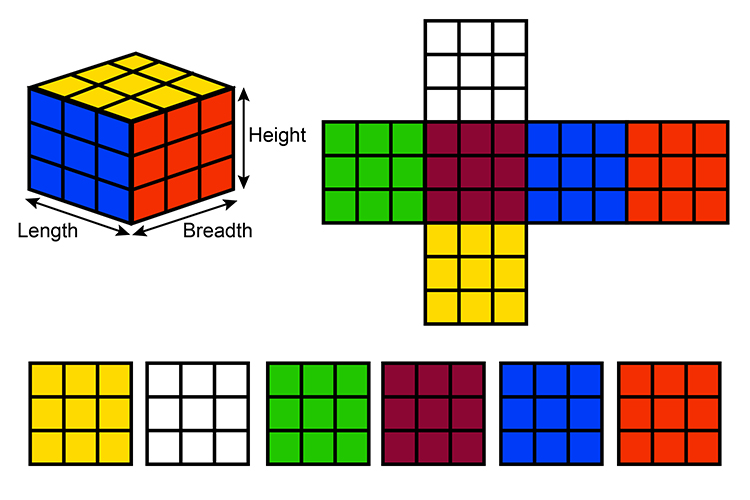# Surface areas formula, cubes, cylinders, pyramids, cones and spheres

## Surface area cube

The surface area of any shape can be found by adding together the surface areas of its parts.

## CubeIf you always imagine pulling apart the cube and drawing it out you can clearly see their six faces.

And if it’s a perfect cube then each face is the same size.

The surface area equals 6 x Area of one face.

Example

An artist wants to make a giant model of a Rubix cube. She purchases six tins of different coloured paint, each of which will cover 10m2 and six sheets of wood 3m x 3m in size. What will be the surface area of the largest cube she can make and will she have enough paint to cover it?

Each face of the cube can have a maximum surface area of 3m\times3m=9m^2
Therefore the maximum surface area the Rubix cube can have is 6\times9m^2 = 54m^2
Each tin of paint will cover 10m2  and each face of the cube = 9m^2
10m^2>9m^2  therefore she will have enough paint.